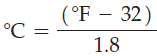×
Get Full Access to Introductory Chemistry - 5 Edition - Chapter 3 - Problem 29q
Get Full Access to Introductory Chemistry - 5 Edition - Chapter 3 - Problem 29q

×

# Solved: The following equation can be used to convert Fahrenheit temperature to CelsiusISBN: 9780321910295 34

## Solution for problem 29Q Chapter 3

Introductory Chemistry | 5th Edition

• Textbook Solutions
• 2901 Step-by-step solutions solved by professors and subject experts
• Get 24/7 help from StudySoup virtual teaching assistantsIntroductory Chemistry | 5th Edition

4 5 1 269 Reviews
26
1
Problem 29Q

The following equation can be used to convert Fahrenheit temperature to Celsius temperature.Use algebra to change the equation to convert Celsius temperature to Fahrenheit temperature.

Step-by-Step Solution:
Step 1 of 3

Solution 29Q

Given : oC =Cross multiplying the above equation, we get :1.8oC = o F - 32o F =  1.8oC + 32

Hence, thè above equation is to convert Celsius temperature to Fahrenheit temperature.

Step 2 of 3

Step 3 of 3

##### ISBN: 9780321910295

Unlock Textbook Solution